# Relativity Tutorial

## Galilean Relativity

Relativity can be described using space-time diagrams. Contrary to popular opinion, Einstein did not invent relativity. Galileo preceded him. Aristotle had proposed that moving objects (on the Earth) had a natural tendency to slow down and stop. This is shown in the space-time diagram below.Note the curved worldline above. This shows a variable velocity, or an acceleration. Galileo objected to Aristotle's hypothesis, and asked what happened to an object moving on a moving ship.Now it is still moving in its final state. Galileo proposed that it is only relative velocities that matter. Thus a space-time diagram can be transformed by painting it on the side of a deck of cards, and then skewing the deck to one side -- but keeping the edges along a straight line:Straight worldlines (unaccelerated particles) remain straight in this process. Thus Newton's First Law is preserved, and non-accelerated worldlines are special. This Galilean transformation does not affect the time. Thus two observers moving with respect to each other can still agree on the time, and thus the distance between two objects, which is the difference in their positions measured at equal times, can be defined. This allowed Newton to describe an inverse square law for gravity.

But Galilean transformations do not preserve velocity. Thus the statement "The speed limit is 70 mph" does not make sense -- but don't try this in court. According to relativity, this must be re-expressed as "The magnitude of the relative velocity between your car and the pavement must be less than 70 mph". Relative velocities are OK.

## Special Relativity

But 200 years after Newton the theory of electromagnetism was developed into Maxwell's equations. These equations describe waves with a speed of 1/sqrt(epsilono*muo), where epsilono is the constant describing the strength of the electrostatic force in a vacuum, and muo is the constant describing the strength of the magnetic interaction in a vacuum. This is an absolute velocity -- it is not relative to anything. The value of the velocity was very close to the measured speed of light, and when Hertz generated electromagnetic waves (microwaves) in his laboratory and showed that they could be reflected and refracted just like light, it became clear that light was just an example of electromagnetic radiation. Einstein tried to fit the idea of an absolute speed of light into Newtonian mechanics. He found that the transformation from one reference frame to another had to affect the time -- the idea of sliding a deck of cards had to be abandoned. This led to the theory of special relativity. In special relativity, the velocity of light is special. Anything moving at the speed of light in one reference frame will move at the speed of light in all unaccelerated reference frames. Other velocities are not preserved, so you can still try to get lucky on speeding tickets.Because the speed of light is special, space-time diagrams are often drawn in units of seconds and light-seconds, or years and light-years, so a unit slope [45 degree angle] corresponds to the speed of light. The set of all light speed world lines going through an event defines the light cones of that event: the past light cone and the future light cone. An example of light cones is shown above. The fancy light picture on the left shows both the past and future light cones of the event where the two worldlines cross, while the schematic version on the right is easy to use in more complicated diagrams.

Thus in the situation shown in 3 space-time diagrams below, the central section shows the worldline of one stationary observer, one observer moving to the right, and two events on the future light cone on the event where the two observers' worldlines cross.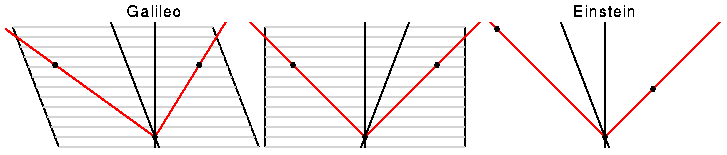The left-hand section of the figure shows the Galilean transformation into the frame of reference of the moving observer. The events on the future light cone have shifted to the left, but they are still at the same time. Since the coordinates x and t just provide a way of describing space-time, and are not the space-time themselves, the two events are still on the future light cone. But now slopes of the light rays have changed, so the speed of light has changed. The Lorentz transformation appropriate for special relativity is shown on the right hand of the figure. The events on the future light cone have shifted to the left as before, but now their times have changed, so the slopes of the light rays do not change. The speed of light is invariant in Einstein's special relativity.

What is the evidence for the invariance of the speed of light? The hypothesis that the speed of light is c relative to its source can easily be disproved by the one-way transmission of light from distant supernovae. When a star explodes as a supernova, we see light coming from material with a large range of velocities dv, at least 10,000 km/sec. Because of this range of velocities, the spectral lines of a supernova are very broad due to the Doppler shift. After traveling a distance D in time D/c, the arrival time of the light would be spread out by dt = (dv/c)(D/c).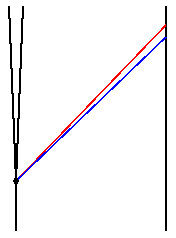However, this DOES NOT happen. For the Crab supernova, with D/c = 6000 years, dv = 10,000 km/sec would give a range of arrival times of 200 years. But the Crab was only bright for 1 year. For very distant supernovae with D/c = 5 billion years, modern observations with spectrographs show that the redshifted and blueshifted light arrives at the same time: within 10 days. This limit on the spread is 5 billion times smaller than the prediction of the "bullet" model of light.

However, light could travel at speed c relative to a medium -- the ether. If it did, then the rate of a "bouncing photon clock" moving with respect to the ether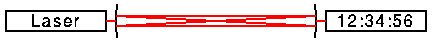would depend on the angle between its photon bouncing axis and its velocity. A stationary bouncing photon clock has a period of P = 2L/c. If it is moving parallel to its axis at velocity v, and light moves at speed c with respect to the ether, then the speed relative to the clock when the photon is moving "upstream" is c-v and the one-way time is L/(c-v). When the photon is moving "downstream" the speed relative to the clock is c+v so the one-way time is L/(c+v). The period is the sum of these times:
```P(par) = L/(c-v) + L/(c+v) = [2L/c]/(1-v2/c2).
```
If the clock is moving perpendicular to its axis, the light has to move a distance L sideways and a distance vt "upstream" to keep up with the clock, where t is the one-way time. The total distance traveled is ct, which is the hypotenuse of a right triangle with sides L and vt. Thus the period is given by:
```(ct)2 = L2 + (vt)2    so   t = L/sqrt(c2-v2)
P(perp) = 2t = [2L/c]/sqrt(1-v2/c2).
```
Thus the ether model predicts that
```dP/P = [P(par)-P(perp)]/P = 0.5*v2/c2.
```
Brillet and Hall (1979, PRL, 42, 549) actually built a bouncing photon clock (a laser stabilized to a Fabry-Perot etalon) on a rotating table and compared its rate to an atomic clock (a laser stabilized to a methane line).The observed dP/P was (1.5 +/- 2.5)*10-15. For the minimum possible velocity of 30 km/sec, due to the orbit of the Earth around the Sun, this is at least a million times smaller than the ether model prediction. The 370 km/sec velocity of the Solar System with respect to the cosmic background radiation gives an ether model prediction 100 million times larger than the Brillet-Hall limit. For this velocity even fourth order effects (v4/c4) can be strongly ruled out. This experiment has been improved by Eisele et al. (2009, PRL, 103, 090401) achieving 10-17 precision which 76 billion times smaller than the ether model prediction.

Michelson and Morley used two bouncing photon clocks at right angles to each other, but without the lasers and counters which didn't exist. This left an L-shaped interferometer. But they were able to show that dP/P was essentially zero instead of the ether model prediction.

The constancy of the speed of light allows the use of radar (RAdio Detection And Ranging) to measure the position and time of events not on an observer's worldline. All that we need are a clock and the ability to emit and detect radar pulses.If we send out a radar pulse at time ts, which is reflected at the event E, and the echo arrives back at time tr, then we know that the light was traveling at c for the entire round-trip journey out to E and back, so the distance of E is D(E) = c*(tr-ts)/2. In our frame of reference we are stationary, and light travels at the same speed coming back from E as it did going out, so the time of the event E is obtained by averaging the send and receive times, t(E) = (tr+ts)/2.

## Time Dilation

Armed with radar, we can determine the time of two events on the worldline of an observer moving with respect to us. We can then compare the time interval we measure to the time interval measured by the moving observer. Consider the two observers A and B below.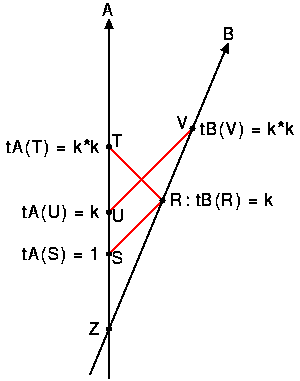They both set their clocks to zero at the event Z where their worldlines cross. A sends out a radar pulse at the event S at time tA(S) = 1. This is received by observer B at event R with time tB(R) = k. The factor k depends on the relative velocity of A and B, but since the light takes some time to travel between A and B, we know that k will be larger than 1. The notation tA means times determined by A, while tB are times determined by B. If A sends out a pulse at some other time, tA = x, it will be received by B at time tB = x*k by the principle of similar triangles. In particular, if A sends out a pulse at time tA = k it will be received by B at time tB = k*k. Now consider the radar pulse reflected by B at event R. It starts at time tB = k. When will A receive it? Since the speed of A with respect to B is the same as the speed of B with respect to A, this time has to be tA(T) = k*k. We can now compute the distance of event R from A's worldline and time of event R according to A, tA(R). These are DA(R) = c(k*k-1)/2 and tA(R) = (k*k+1)/2. Thus the speed of B according to A is
```v = D/t = DA(R)/tA(R) = c(k*k-1)/(k*k+1).
```
We can solve for k giving
```k = sqrt((1+v/c)/(1-v/c))
```
which is the relativistic Doppler shift formula. But we also find that tA(R) > tB(R), so A says that B's clock is running slow. The amount of this time dilation is
```(1+k*k)/(2*k) = 1/sqrt(1-v2/c2).
```
Thus moving clocks run slow. Note that B will also find that A's clock is running more slowly than his. There is a symmetric disagreement about clock rates. I have prepared an animation that toggles back and forth from the point-of-view of observer A to the point-of-view of observer B and illustrates the symmetry.

This slow down factor is exactly the slow down calculated above in the ether model for a bouncing photon clock moving perpendicular to its bounce axis. The clock moving parallel to the axis slows down by the same amount under special relativity because of the Lorentz-Fitzgerald contraction of moving objects in the direction of motion.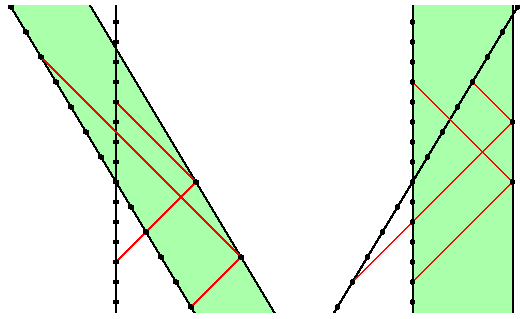The space-time diagrams above both show a rod moving past an observer. On the left the rod is moving, while on the right the same situation is shown in the rod's frame of reference. The observer moving with respect to the rod makes a radar determination of its length, as does an observer moving along with the rod. The observer on the rod sees a length of 5 light-ticks because it takes 10 ticks for light to make the round trip to the end of the rod and back. The observer moving with respect to the rod at v = 0.6*c measures only 8 ticks for the round trip and thus gives the length of the rod as 4 light-ticks. Thus the length of a moving rod appears to be reduced by a factor of sqrt(1-v2/c2). Thus length contraction changes P(par) for the bouncing photon clock to
```P(par) = [2L*sqrt(1-v2/c2)/c]/(1-v2/c2)
= [2L/c]/sqrt(1-v2/c2) = P(perp)
```
so the rate of a bouncing photon clock does not depend on the angle between its velocity and its bouncing axis.

Because the clocks of different observers run at different rates, depending on their velocities, the time for a given observer is a property of that observer and his worldline. This time is called the proper time because it is "owned" by a given particle, not because it is the "correct" time. Proper time is invariant when changing reference frames because it is the property of a particle, not of the reference frame or coordinate system. In general, given any two events A and B with B inside the future light cone of A, there is one unaccelerated worldline connecting A and B, just as there is one straight line connecting two points in space. In the frame of reference of the observer following this unaccelerated worldline, his clock is always stationary, while clocks following any other worldline from A to B will be moving at least some of the time. Because moving clocks run slow, these observers will measure a smaller proper time between events A and B than the unaccelerated observer. Thus the straight worldline between two events has the largest proper time, and all other curved worldlines connecting the two events have smaller proper times. This is exactly analogous to the fact that the straight line between two points has the smallest length of all possible curves between the points. Thus the "twin paradox" is no more paradoxical than the statement that a man who drives straight from LA to Las Vegas will cover fewer miles than a man who drives from LA to Las Vegas via Reno.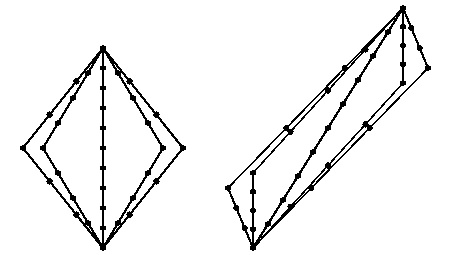The pair of space-time diagrams above show quintuplets separated at birth. The middle worldline shows the quint who stays home. The space-time diagram on the left is done from the point of view of the middle quint. Each dot on a worldline is a birthday party, so the middle quint is 10 years old when they all rejoin each other, while the other quints are 6 and 8 years old. The space-time diagram on the right shows the same events from the point of view of an observer initially moving with one of the moving quints. When the quints come together their ages are still 6, 8, 10, 8, and 6 years. Thus the straight worldline between two events can be found by maximizing the proper time, just as the straight line between two points can be found by minimizing the length.

## General Relativity

Now we come to a matter of gravity: how can gravity be an inverse square law force, when the distance between two objects can not even be defined in Einstein's special relativity? Special relativity was constructed to satisfy Maxwell's equations, which replaced the inverse square law electrostatic force by a set of equations describing the electromagnetic field. So gravity was the only remaining action-at-a-distance inverse square law force. And gravity has a unique property; the acceleration due to gravity at a given place and time is independent of the nature of the body.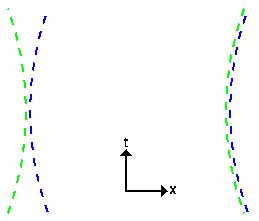The space-time diagram above shows a proton and an antiproton moving under the influence of an electric field on the left and a gravitational field on the right. Gravity accelerates all objects equally. This fact was known to Newton, and tested to an accuracy of 1 part in 100 million by Eotvos. Later work by Dicke and by Braginsky has improved the accuracy of the test to 1 part in a trillion. A space mission called
STEP has been proposed which will test the equality of acceleration to 1 part per quintillion. In 2000 STEP was selected for further study in NASA's SMEX program. STEP did not proceed, but a different satellite was launched in 2016, and hopes to achieve 1 part per quadrillion accuracy.

Thus through any event in space-time, in any given direction, there is only one worldline corresponding to motion solely influenced by gravity. Compare this to the geometric fact that through any point, in any given direction, there is only one straight line. We are led to propose that worldlines influenced only by gravity are really straight worldlines. But how can an accelerating body have a straight worldline? It all depends on how you measure it. Suppose we plot a straight line on polar graph paper, and then make a plot of radius vs angle as shown below?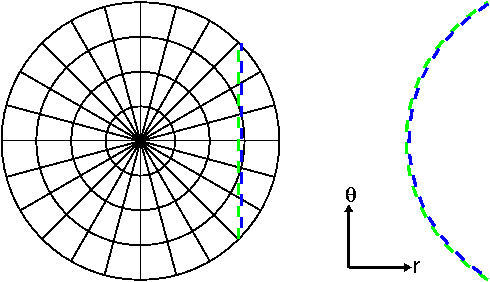In the radius vs theta plot the straight line is curved. I have shown adjacent two lines dashed and labeled just like the proton and antiproton shown earlier to emphasize that while there are an infinite number of curved lines in the radius vs theta plot, there is only one straight line through the initial point with the initial direction.

## Principle of Equivalence

Einstein proposed that the effects of gravity (in a small region of spacetime) are equivalent to the effect of using an accelerated frame of reference without gravity. As as example, consider the famous "Einstein elevator" thought experiment. If an elevator far out in space accelerates upward at 10 meters/second2, it will feel like a downward acceleration of gravity at 1 g = 10 m/s2. If a clock on the ceiling of the elevator emits flashes of light f times per second, an observer on the floor will see them arriving faster than f times per second because of the Doppler shift due to the acceleration of the elevator during the light transit time.The space-time diagram on the left above shows the clock on the elevator ceiling emitting flashes of light. The light transit time is h/c where h is the height of the ceiling, and the velocity change is a*h/c so the Doppler shift increases the rate of flash arrival by a factor of (1+a*h/c2), so the flash arrival rate is f' = (1+a*h/c2)*f. On the right is the same situation with stationary clocks in a gravitational field. In order to have the flash arrival rate faster by a factor of (1+g*h/c2), the clock on the ceiling must run faster by this factor. In other words, clocks run faster when they are high up in a gravitational field. This effect has been seen in the laboratory by Pound and Rebka (1960, PRL, 4, 337) who used the Mossbauer effect to measure a frequency shift (f'/f -1) = (2.57+/-0.20)*10-15 after dropping photons a distance of 22.6 meters. The expected shift was 2.46*10-15.

The effect of gravity on clocks was tested to greater precision by Vessot etal (1980, PRL, 45, 2081) who launched a hydrogen maser straight up at 8.5 km/sec, and watched its frequency change as it coasted up to 10,000 km altitude and then fell back to Earth. The frequency shift due to gravity was (f'/f -1) = 4*10-10 at 10,000 km altitude, and the experimental result agreed to within 70 parts per million of this shift.

Because of the gravitational speedup for uphill clocks, an observer moving between two events can achieve a larger proper time by shifting his worldline upward in the middle. Going too far upward requires moving so fast that time dilation due to motion reduces the proper time more than the gravitational speedup, so there is an optimum curvature to the worldline that maximizes the proper time.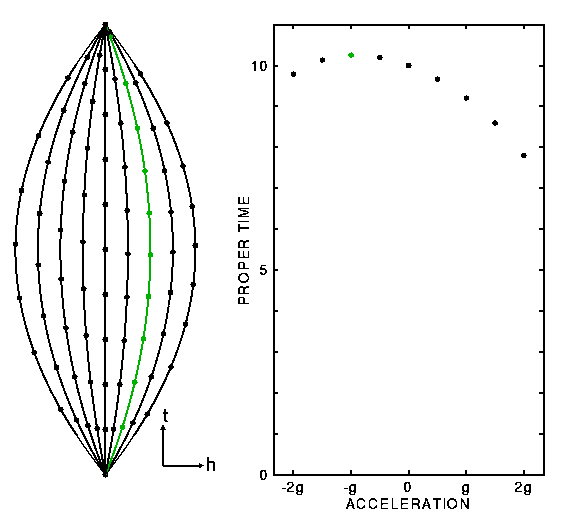The space-time diagram on the left above shows 9 observers moving between two events with different accelerations. The third from the right has the correct balance between going uphill to get a faster clock rate and avoiding motion to avoid time dilation. As a result, this observer has the largest proper time between the two events. Note that the accelerations are negative for paths that have a maximum height, so the third worldline from the right is plotted as the third dot from the left on the chart. The optimum worldline curvature is the acceleration of gravity, and it is negative because things fall down, not up.

## Curved Spacetime

Curved coordinates alone, such as the polar graph, do not provide a satisfactory model for gravity. Two straight lines through the same point but with different directions will never cross again, while two worldlines influenced only by gravity which pass through the same event with different velocities can cross again. Consider the Galileo spacecraft, which made two Earth flybys. In between the flybys, Galileo was on an elliptical orbit with a 2 year period. In order to allow "straight" lines to cross multiple times, a curved space-time is needed. As a familiar example of a curved space, consider the surface of the Earth and the great circle arc connecting two cities. The great circle is the shortest distance between two points on the surface of the Earth, and it is the path followed by airliners.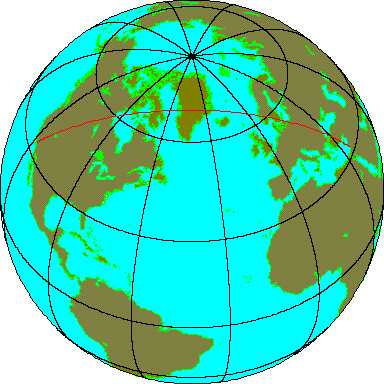The great circle path from Los Angeles (34 N, 118 W) to Tel Aviv (32 N, 35 E) goes all the way to 70 N latitude.

Plotting latitude vs longitude, as if longitude were time and latitude position, gives the pseudo-spacetime diagram below.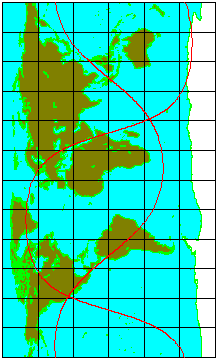The two great circles through Los Angeles, one to Tel Aviv and one to Singapore, are both "straight" lines, but they intersect in two places. This is impossible in plane geometry but it does occur in non-Euclidean geometry. The pseudo-spacetime diagram above is almost identical to a real spacetime diagram for objects moving in a tunnel drilled through the center of a massive sphere. Gravity produces oscillatory motions so worldlines for different objects, each influenced only by gravity, can cross at many events.

Einstein was able to compute the perihelion advance of Mercury using general relativity, and his calculation matched an observed discrepancy with Newtonian predictions. Einstein also computed that light passing by the Sun would be deflected by twice as much as a prediction using Newtonian gravity and Newton's particle model for light would suggest. The same effect causes a delay of light passing by the limb of the Sun, known as the Shapiro delay, which has now been measured to great accuracy and agrees with the prediction of general relativity.

## Light cones in curved space-time

Unlike the restricted set of Lorentz transformations allowed in special relativity, the more general coordinate transformations of general relativity will change the slope of the walls of the lightcones. In other words, the speed of light (dx/dt) will change in the transformed coordinates: dx'/dt' will not equal dx/dt in general. The light cones can tilt or stretch. The figure below shows "lightcones" added to the radius vs angle example given above:The proper generalization of the special relativistic rule that velocities of objects should be less than c is that the worldlines of objects should be inside the lightcones, but the lightcones can tilt over or stretch vertically or horizontally. It is quite possible for a stationary object to have a worldline that is outside the lightcone. This happens inside the stationary limit of a black hole, for example, or for distances greater than the Hubble radius in cosmological models, as shown below in a space-time diagram from my Cosmology Tutorial.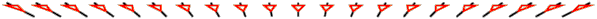Thus the fundamentals of relativity that are important for cosmology are:

• The speed of light is a constant independent of the velocity of the source or the observer.
• Events that are simultaneous as seen by one observer are generally not simultaneous as seen by other observers, so there can be no absolute time.
• Each observer can define his own proper time -- the time measured by a good clock moving along his worldline.
• Observers can assign times and positions to events not on their worldlines using radar observations.
• Every observer will see his clock running faster than other clocks which are moving with respect to him, and this is a mathematically consistent pattern required by the properties of radar observations.
• As a result, the unaccelerated worldline between two events will have the longest proper time of all worldlines connecting these events.
• In the presence of gravity, the worldlines of objects accelerated only by gravity have the longest proper times.
• Gravity requires that spacetime have a non-Euclidean geometry, and this curvature of spacetime must be created by matter.

Relativity also leads to interesting objects such as black holes, but these are not very relevant to cosmology.

There are many books on relativity available, but two that stick to a simple level of mathematics are:

For (much) more online information about gravity and space-time, see John Baez's General Relativity Tutorial or the relativity sections of the Physics FAQ.
Andrew Hamilton's Home Page has simulations of what you would see while in orbit around a black hole, or while falling in.
J. W. Hinson's FAQ has information about relativity and faster than light travel.
Chris Hillman has deleted his relativity web pages including his partial list of incorrect refutations of General Relativity, but you can see archived versions at web.archive.org by entering the URL "www.math.ucr.edu/home/baez/RelWWW/HTML/wrong.html" into their Wayback machine.